﻿ 个性化信息推荐方法研究
«上一篇文章快速检索 高级检索

 智能系统学报2018, Vol. 13Issue (2): 189-195  DOI: 10.11992/tis.2017010020

### 引用本文JIANG Xinjing, QI Xiaogang, LIU Lifang. Research on the recommendation method of personalized information[J]. CAAI Transactions on Intelligent Systems, 2018, 13(2): 189-195. DOI: 10.11992/tis.201701002.### 文章历史

Research on the recommendation method of personalized information
JIANG Xinjing, QI Xiaogang, LIU Lifang
School of mathematics and statistics, Xi dian University, Xi’an 710071, China
Abstract: It’s an excessively informational and more fragmented era that is contributed to the development of information technology and the Internet. At present, personalized recommendation is a relatively effective way to help users gain various network information. Recommendations may not be ideal as the traditional algorithms rarely focus on the fast speed of information updating and change of users interests. We propose a combined recommendation algorithm by introducing an interception factor and calls it the CR algorithm. The core idea of it is to introduce the interception factor to the content-based recommendation algorithm and user-based collaborative filtering algorithm. The mixed recommendation consists of the content-based recommendation algorithm and user-based collaborative filtering algorithm. Recommending results of CR algorithm are divided into the outcomes produced by mixed recommendation algorithm and the user-based collaborative filtering algorithm. It is the publishing time of information that decides which algorithm should be chosen to produce recommendations: the mixed recommendation algorithm is selected when the difference between browsing time and message publishing time does not exceed some threshold, or directly chooses the user-based collaborative filtering. Simulation results based on real data show the algorithm we proposed is superior to other existing algorithms.
Key words: network information    interception factor    information push    content-based recommendation    behavior-based similarity collaborative filtering    content-based similarity collaborative filtering    mixed recommendation    combined recommendation

1 个性化推荐方法 1.1 问题定义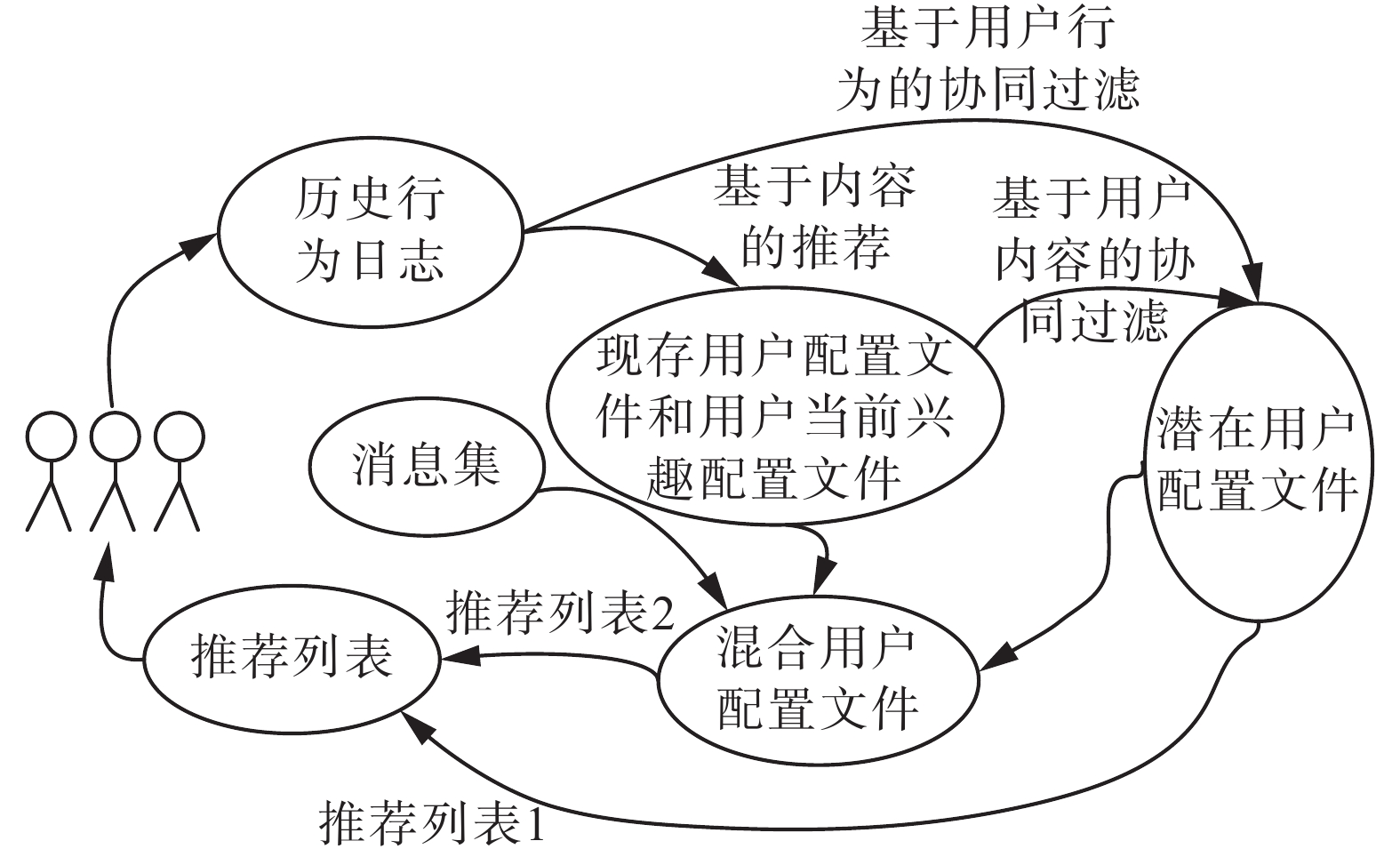Download: 图 1 方案框架 Fig. 1 Scheme framework
1.2 现存用户配置文件

1.2.1 向量空间模型

 ${w_{ij}} = tf\left( {i,j} \right) \times \log \left[ {1 + n/n\left( j \right)} \right]/\max {{Other}}\left( {i,j} \right)$ (1)

1.2.2 用户现存配置文件、时间因子以及用户当前兴趣配置文件

 ${u_{i + 1}} = 1/\left( {1 + \alpha \left| {{t_{t + 1}} - {t_i}} \right|} \right)$ (2)

1)　 ${{{UCF}}_u} \,=\, \left( {{{{wc}}_{u1}},{{{wc}}_{u2}}, \cdots ,{{{wc}}_{ul}}} \right)$ , ${{{UCF}}_{{us}}} \,=\, \left( {{{{wc}}_{{u^s}1}},} \right.$ $\left. {{{{wc}}_{{u^s}2}}, \cdots ,{{{wc}}_{{u^s}l}}} \right)$

2)　for each ${k_j} \in K$ do

3)　　 ${{{wc}}_{uj}} = 0$ , ${{{wc}}_{{u^s}j}} = 0$

4)　end for

5)　for each ${f_{ui}} \in {F_{{us}}}$ do

6)　　if $i = = s$ then

7)　　　 ${{{wc}}_{{u^s}j}} = {w_{uij}}$

8)　　else

9)　　　 ${\mu _i} = \displaystyle\frac{1}{{1 + \alpha \left| {{t_i} - {t_{i - 1}}} \right|}}$

10)　　　 ${{{wc}}_{{u^s}j}} = {\mu _i} * {{{wc}}_{{u^s}j}} + {w_{uij}}$

11)　　end for

12)　end for

13)　for each ${f_{ui}} \in {F_u}$ do

14)　　if $s = = {n_u}$ then

15)　　　 ${{{wc}}_{ui}}{{ = }}{w_{uij}}$

16)　　else

17)　　　 ${\mu _i} = \displaystyle\frac{1}{{1 + \alpha \left| {{t_i} - {t_{i - 1}}} \right|}}$

18)　　　 ${{{wc}}_{uj}}{{ = }}\mu *{{{wc}}_{uj}} + {w_{uij}}$

19)　　end if

20)　end for

1.3 潜在配置文件

1.3.1 混合相似性的计算

 ${{simAct}}\left( {u,v} \right) = \left| {{F_{us}} \cap {F_v}} \right|/\sqrt {\left| {{F_{us}}} \right| \times \left| {{F_u}} \right|}$ (3)
 ${{simCon}}\left( {u,v} \right) = \left( {{{{CUF}}_{us}} \cdot {{{CUF}}_v^{{T}}}} \right)/\sqrt {\left| {{{{CUF}}_{us}}} \right| \times \left| {{{{CUF}}_v}} \right|}$ (4)

 ${{sim}}\left( {u,v} \right) = \beta \times {{simAct}}\left( {u,v} \right) + \left( {1 - \beta } \right) \times {{simCon}}\left( {u,v} \right)$ (5)

1)　 ${{numSimNews}} \,=\, 0$ ${{innerPro}} \,=\, 0$ ${{{norm}}_u} \,=\, 0$ ${{{norm}}_v} = 0$

2)　for each ${f_{ui}} \in {F_{us}}$ do

3)　　for each ${f_{uj}} \in {F_v}$ do

4)　　　if ${f_{ui}} = {f_{uj}}$ then

5)　　　　 ${{numSimNews}} = 1 + {{numSimNews}}$

6)　　　end if

7)　　end for

8)　end for

9)　 ${{simAct}}(u,v) \,\,=\,\, {{numSimNews}}/{{sqrt}}({{len}}({F_u})\,\,\,*$ ${{len}}({F_v}))$

10)　for each $w{c_{{u^s}j}} \in {{{UCF}}_{us}}$ do

11)　　 ${{innerPro}} = {{innerPro}} + {{{wc}}_{{u^s}j}}*{{{wc}}_{vj}}$

12)　　 ${{{norm}}_{us}} = {{{norm}}_u} + {{{wc}}_{{u^s}j}}*{{{wc}}_{{u^s}j}}$

13)　　 ${{{norm}}_v} = {{{norm}}_v} + {{{wc}}_{vj}}*{{{wc}}_{vj}}$

14)　end for

15)　 ${{simCon}}(u,v) \,\,=\,\, {{innerPro}}/({{sqrt}}({{{norm}}_{us}})\,\,*\,\,\,{{sqrt}}$ $({{{norm}}_v}))$

16)　 ${{sim}}\left( {u,v} \right)\!{{ = }}\! \beta \!*\!{{simAct}}\left( {u,v} \right) \!+\! \left( {1 \!\!-\! \beta } \right)\!*\!{{simCon}}\left( {u,v} \right)$

1.3.2 潜在用户配置文件和相似用户文件的生成

 ${{{wm}}_{uj}} = \sum\nolimits_{{v_i}j} {\left[ {{{{wc}}_{{v_i}j}} \times {{sim}}\left( {u,{v_i}} \right)/\sum\nolimits_{{v_a} \in {U_u}} {{{sim}}\left( {u,{v_a}} \right)} } \right]}$ (6)

1)　 ${{sumSim}} = 0$ ${{{UMF}}_u} = \left( {{{{wm}}_{u1}},{{{wm}}_{u2}}, \cdots ,{{{wm}}_{ul}}} \right)$

2)　for each ${k_j} \in K$ do

3)　　 ${{{wm}}_{ij}} = 0$

4)　end for

5)　for each ${v_a} \in {U_u}$ do

6)　　 ${{sumSim}} = {{sumSim}} + {{sim}}\left( {u,{v_a}} \right)$

7)　end for

8)　for each ${v_i} \in {U_u}$ do

9)　　for each ${k_j} \in K$ do

10)　　　 ${{{wm}}_{uj}} = {{{wm}}_{uj}} + {{{wc}}_{{v_i}j}} * {{sim}}\left( {u,{v_i}} \right)/{{sumSim}}$

11)　　end for

12)　end for

1.4 用户混合配置文件的生成

 ${{{wb}}_{uj}} = \gamma {{{wc}}_{{u^s}j}} + \left( {1 - \gamma } \right){{{wm}}_{uj}}$ (7)

1)　 ${{{UBF}}_u} = \left( {{{{wb}}_{u1}},{{{wb}}_{u2}}, \cdots ,{{{wb}}_{ul}}} \right)$

2)　for each ${k_i} \in K$ do

3)　　 ${{{wb}}_{uj}} = 0$

4)　　 ${{{wb}}_{uj}} = \gamma {{{wc}}_{uj}} + (1 - \gamma ){{{wm}}_{uj}}$

5)　end for

1.5 推荐结果的生成

${l_1}$ 部分由混合配置文件生成，即通过添加时间因子 ${\varepsilon _1}$ 来限定消息是否采用混合推荐方法：当消息的发布时间与当前时间的时间间隔小于 ${\varepsilon _1}$ ，若满足，则该文件采用混合推荐方法，否则将不采用。详细过程如下：

 ${t_{cur}} - {t_0} \leqslant {\varepsilon _1}$ (8)

 ${d_0} \cdot {\bf{BUF}}_u^{{T}} \geqslant {\varepsilon _2}$ (9)

${l_2}$ 部分直接由基于内容相似和行为相似的协同过滤算法生成。详细过程如下：

 ${w_{u{d_0}}} = \sum\nolimits_{j = 1}^h {{w_{{v_j}{d_0}}}} * {{sim}}\left( {u,{v_j}} \right)$ (10)

2 实验和分析

 $F = \frac{1}{{1/{{reacll}} + 1/{{precision}}}}$ (11)

 ${{recall}} = \frac{{\sum\nolimits_{{u_i} \in U} {{{hit}}\left( {{u_i}} \right)} }}{{\sum\nolimits_{{u_i} \in U} {T\left( {{u_i}} \right)} }}$ (12)

 ${{precision}} = \frac{{\sum\nolimits_{{u_i} \in U} {{{hit}}\left( {{u_i}} \right)} }}{{\sum\nolimits_{{u_i} \in U} {L\left( {{u_i}} \right)} }}$ (13)表 1 recall与 $\,{\beta}$ 的关系 Tab.1 Relationship between recall and $\,{\beta}$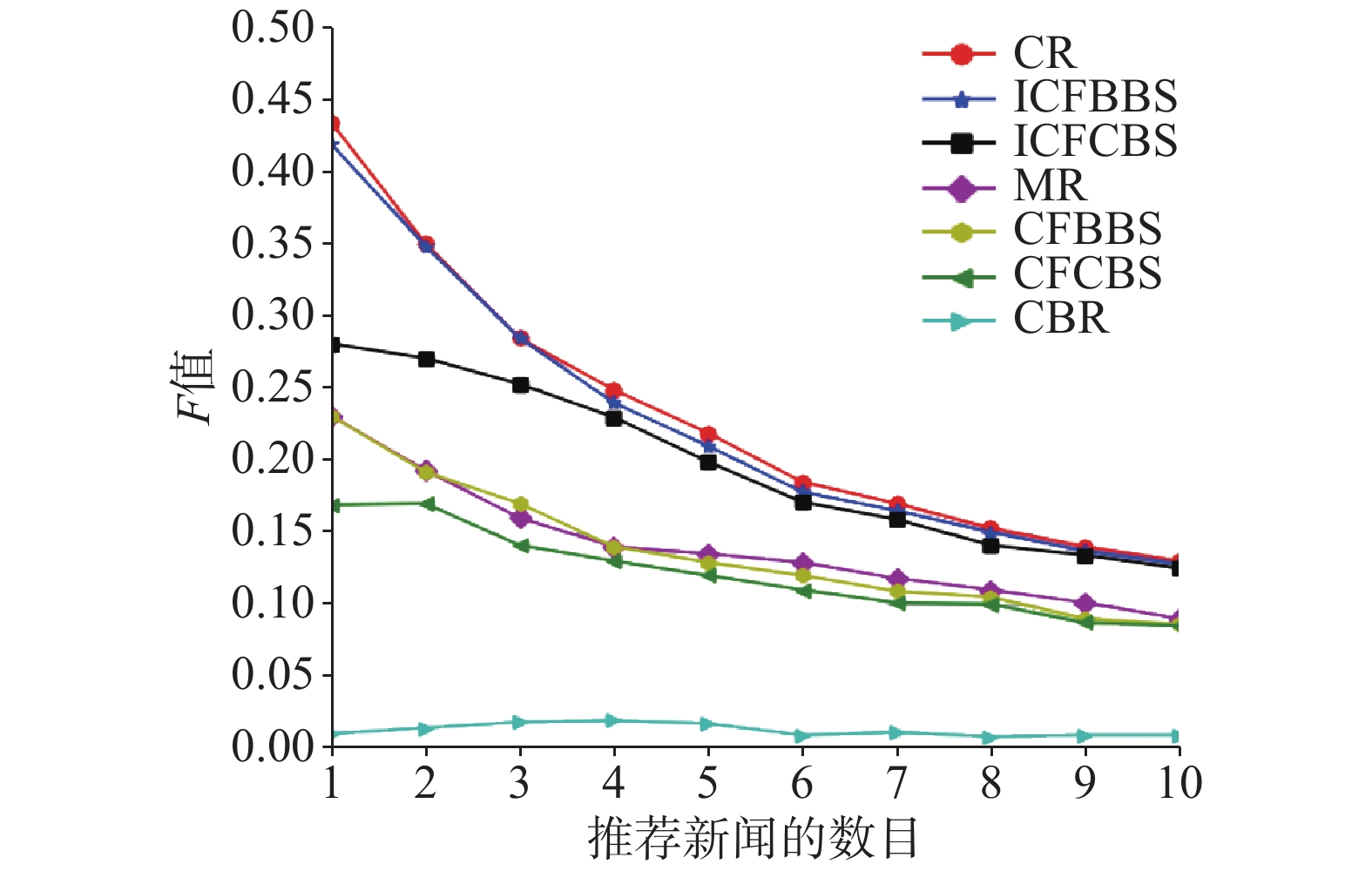Download: 图 2 F值比较 Fig. 2 Comparison of F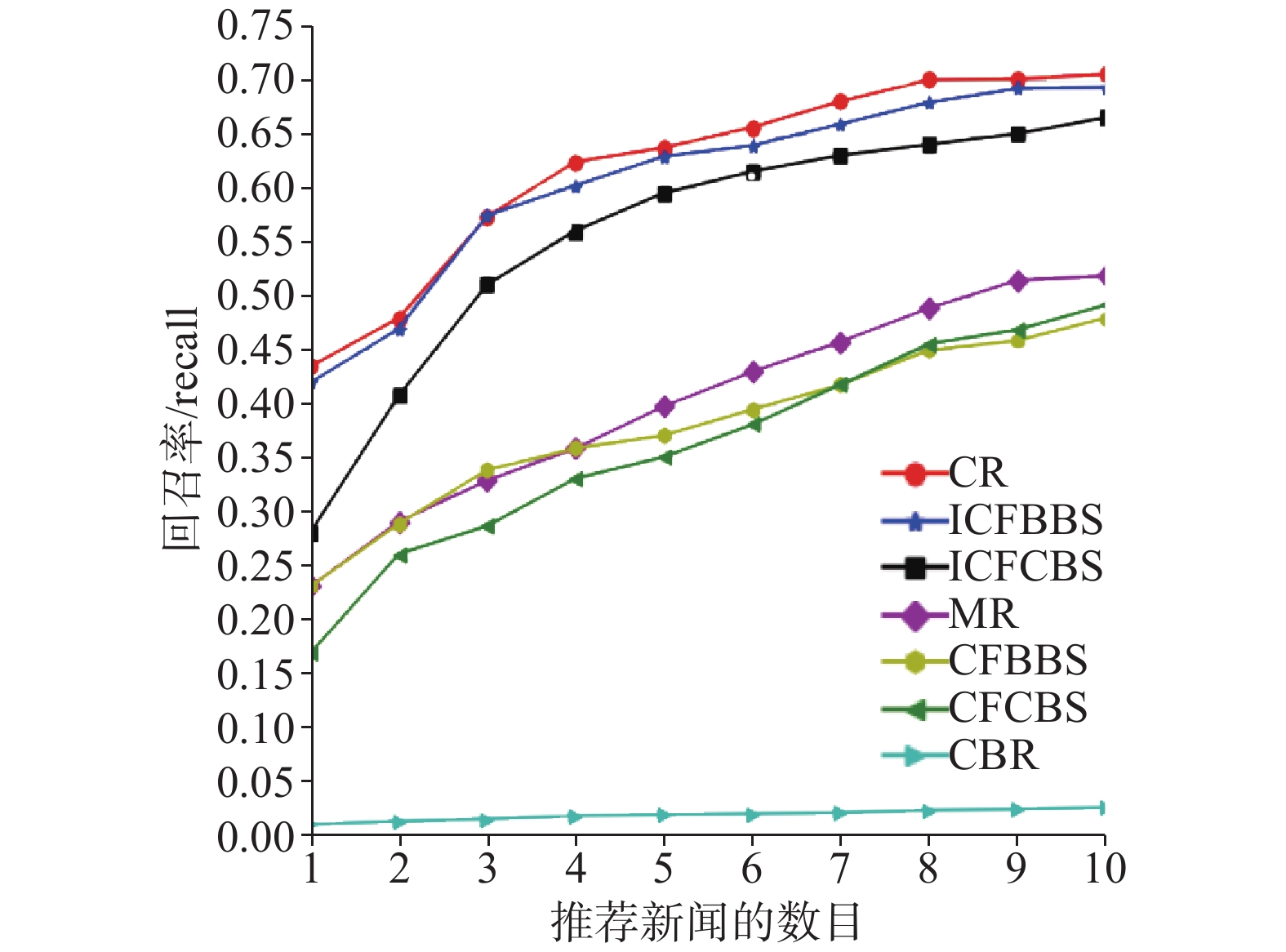Download: 图 3 召回率比较 Fig. 3 Comparison of recall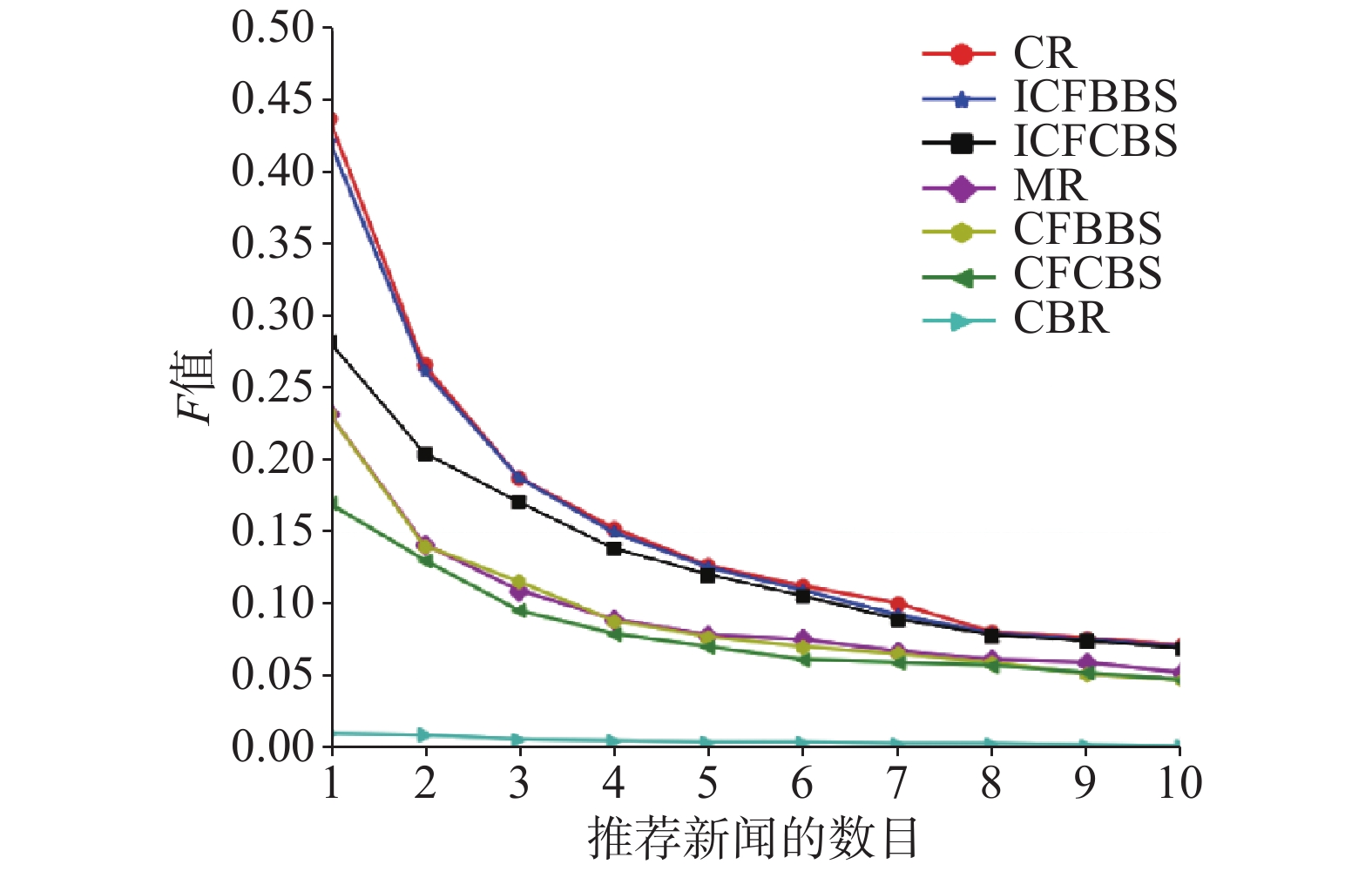Download: 图 4 精确度比较 Fig. 4 Comparison of precision

 ${{Diversity}} = 1 - \frac{{\sum\nolimits_{i,j \in R\left( u \right),i \ne j} {s\left( {i,j} \right)} }}{{0.5\left| {R\left( u \right)} \right|\left( {\left| {R\left( u \right)} \right| - 1} \right)}}$ (14)

 ${{Diversity}} = \frac{1}{{\left| U \right|}}\sum\limits_{u \in U} {{{Diversity}}\left( {R\left( u \right)} \right)}$ (15)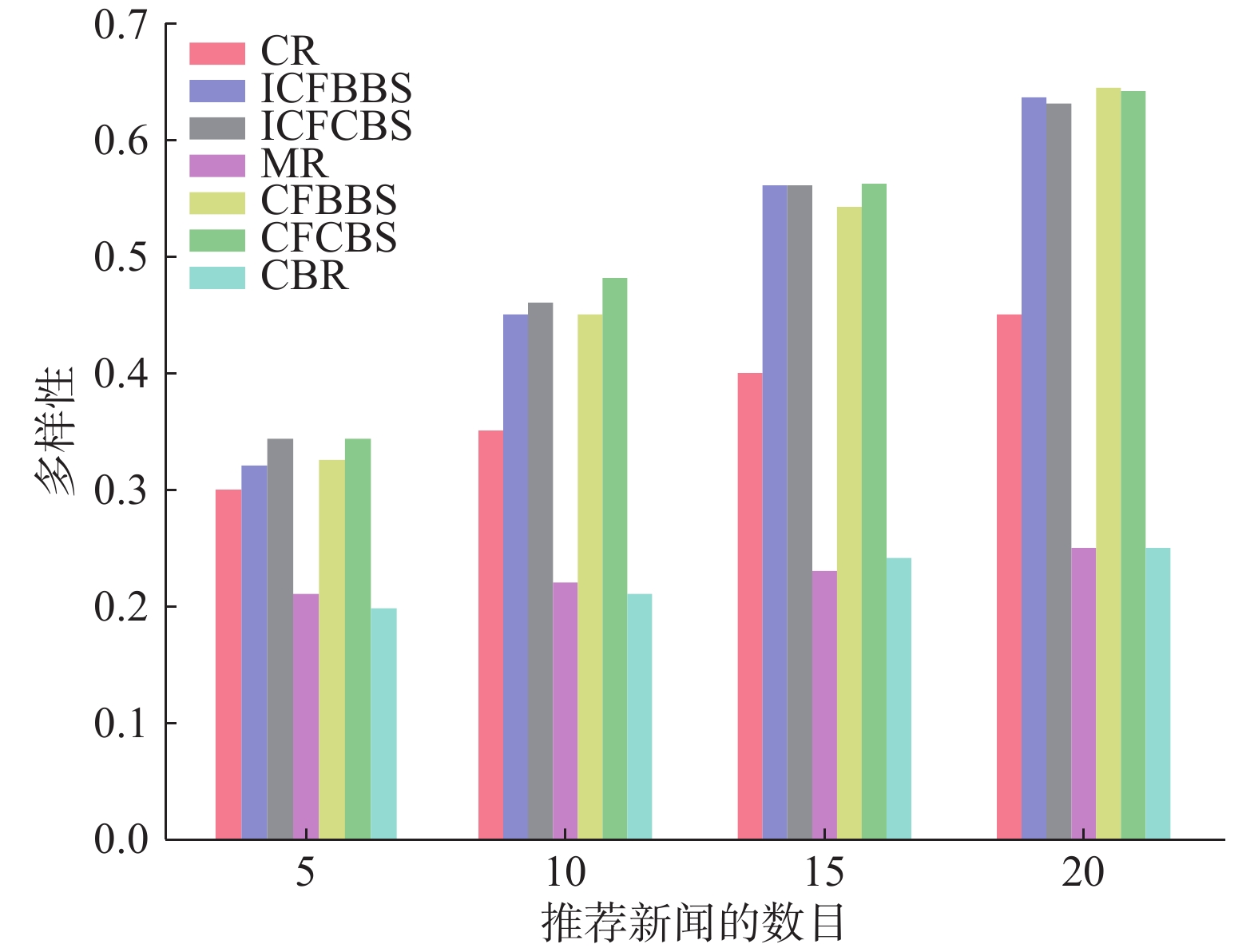Download: 图 5 多样性比较 Fig. 5 Comparison of diversity

3 结束语

  李佳珊. 个性化新闻推荐引擎中新闻分组聚类技术的研究与实现[D]. 北京: 北京邮电大学, 2013. LI Jiashan. Research and implementation of text clustering for personalized news recommandation system[D]. Beijing: Beijing University of Posts and Telecommunications, 2013. (0)  项亮. 推荐系统实践[M]. 北京: 人民邮电出版社, 2012. (0)  BALABANOVIĆ M, SHOHAM Y. Fab: content-based, collaborative recommendation[J]. Communications of the ACM, 1997, 40(3): 66-72. DOI:10.1145/245108.245124 (0)  MANDL M, FELFERNIG A, TEPPAN E, et al. Consumer decision making in knowledge-based recommendation[J]. Journal of intelligent information systems, 2011, 37(1): 1-22. DOI:10.1007/s10844-010-0134-3 (0)  LI Xiaohui, MURATA T. A knowledge-based recommendation model utilizing formal concept analysis and association[C]//Proceedings of the 2nd International Conference on Computer and Automation Engineering. Singapore, 2010: 221–226. (0)  GARCIN F, ZHOU Kai, FALTINGS B, et al. Personalized news recommendation based on collaborative filtering[C]//Proceedings of the 2012 IEEE/WIC/ACM International Joint Conferences on Web Intelligence and Intelligent Agent Technology. Washington, DC, USA: IEEE, 2012: 437–441. (0)  DARVISHY A, IBRAHIM H, MUSTAPHA A, et al. New attributes for neighborhood-based collaborative filtering in news recommendation[J]. Journal of emerging technologies in web intelligence, 2015, 7(1): 13-19. (0)  YANG Wu, TANG Rui, LU Ling. A fused method for news recommendation[C]//Proceedings of the 2016 International Conference on Big Data and Smart Computing (BigComp). Hong Kong, China, 2016: 341–344. (0)  LU Zhongqi, DOU Zhicheng, LIAN Jianxun, et al. Content-based collaborative filtering for news topic recommendation[C]//Proceedings of the 29th AAAI Conference on Artificial Intelligence. Austin, Texas, USA, 2015: 217–223. (0)  LIU Y, BAO L, GAO L. Trust-based new recommendation algorithm of collaborative filtering combination[J]. Information Japan, 2013, 16(7): 4555-4576. (0)  WANG Jingjin, LIN Kunhui, LI Jia. A collaborative filtering recommendation algorithm based on user clustering and slope one scheme[C]//Proceedings of the 2013 8th International Conference on Computer Science & Education (ICCSE). Colombo, Sri Lanka, 2013: 1473–1476. (0)  CAPELLE M, FRASINCAR F, MOERLAND M, et al. Semantics-based news recommendation[J]//Proceedings of the 2nd International Conference on Web Intelligence, Mining and Semantics. Craiova, Romania, 2012: 27. (0)  CUI Limeng, SHI Yong. A Method based on one-class SVM for news recommendation[J]. Procedia computer science, 2014, 31: 281-290. DOI:10.1016/j.procs.2014.05.270 (0)  REN Rui, ZHANG Lingling, CUI Limeng, et al. Personalized financial news recommendation algorithm based on ontology[J]. Procedia computer science, 2015, 55: 843-851. DOI:10.1016/j.procs.2015.07.151 (0)  LOMMATZSCH A, KENTER T, DE VRIES A P, et al. Real-time news recommendation using context-aware ensembles[M]//DE RIJKE M. Advances in Information Retrieval. Cham, Germany: Springer, 2014. (0)  杨博, 赵鹏飞. 推荐算法综述[J]. 山西大学学报: 自然科学版, 2011, 34(3): 337-350. YANG Bo, ZHAO Pengfei. Review of the art of recommendation algorithms[J]. Journal of Shanxi university: natural science edition, 2011, 34(3): 337-350. (0)  路永和, 李焰锋. 改进TF—IDF算法的文本特征项权值计算方法[J]. 图书情报工作, 2013, 57(3): 90-95. LU Yonghe, LI Yanfeng. Improvement of text feature weighting method based on TF-IDF algorithm[J]. Library and information service, 2013, 57(3): 90-95. (0)